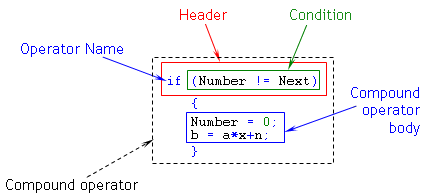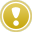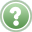English

MQL4 BookBasics of MQL4Operators

# Operators

## The term of operator

One of the key notions of any programming language is the term of operator. Coding seems to be impossible for the person that has not completely learned this term. The sooner and more properly a programmer learns what operators are and how they are applied in a program, the sooner this programmer starts writing his or her own programs.

An operator is a part of a program; it is a phrase in an algorithmic language that prescribes a method of data conversion.

Any program contains operators. The closest analogy to operator is a sentence. Just as normal sentences compose the text of a story, so operators compose a program.

## Properties of operators

There are two kinds of properties of operators: a common property, and specific properties of different operators.

### Common property of operators

All operators have one common property; they all are executed.

We can say that an operator is an instruction containing the guide to operations (the description of an order). For a computer, executing a program running on it means consecutively passing from one operator to another, while complying with the orders (prescriptions, instructions) contained in the operators.

An operator is just a record, a certain sequence of characters. An operator does not have any levers, wires, or memory cells. This is why, when a computer is executing a program, nothing happens in operators as such, they continue staying in the program as composed by the programmer. However, the computer, which has all those memory cells and links between the cells, experiences all the transformations inside. If your PC has executed some transformations of data according to the instruction contained in an operator, you say that the operator has been executed.

### Specific properties of operators

There are several kinds of operators. Operators of each type have their specific properties. For example, the property of an assignment operator is its ability to assign a certain value to the given variable; the property of a loop operator is its multiple executions and so on. Specific properties of operators are considered in detail in the corresponding sections of the chapter on operators in this book. We will only say here that all types of operators have their own properties that are typical only for their type and are not repeated in any other types.

## Types of operators

There are two types of operators: simple operators and compounds.

### Simple operators

Simple operators in MQL4 end with the character ";" (semicolon). Using this separator, the PC can detect where one operator ends and another one starts. A semicolon is as necessary in a program as a period is necessary in a normal text to separate sentences. One operator may take several lines. Several operators may be placed in one line.Every simple operator ends with character ";" (semicolon).

Here are examples of simple operators.

```   Day_Next= TimeDayOfWeek(Mas_Big[n]+60);   // Simple operator
Go_My_Function_ind();                        // Simple operator
a=3;  b = a * x + n;  i++;                   // Several operators in a line
Print("   Day= ",TimeDay(Mas_Big[s]),     // One operator..
"   Hour=",TimeHour(Mas_Big[s]),    // is located..
"   Minute=",TimeMinute(Mas_Big[s]),// in several ..
"   Mas_Big[s]= ",Mas_Big[s],    // lines
"   Mas_Big[s]= ",Mas_Big[s]);```

### Compound operators (compounds)

A compound operator consists of several simple ones separated by character ";" and is enclosed in braces. In order to be able to use several operators where only one is expected to be located, programmers use a compound operator (they also call it "block" or "block of code"). The list of operators in a compound is separated by braces. The presence of a closing brace marks the end of a compound operator.Here is an exemplary use of a compound in a conditional operator. It starts with the conditional operator if(expression) followed by a compound. The compound operator contains a list of executable operators.Figure 17 Compound operator.The body of a compound operator is enclosed in braces. Every compound operator ends with a closing brace.

Here are examples of compound operators.

```                                            // Example of operator switch
switch(ii)                                  // Operator switch(expression)
{                                        // Opening brace
case 1: Buf_1[Pok-f+i]= Prognoz;  break; // Nested operators (operator body)
case 2: Buf_2[Pok-f+i]= Prognoz;  break; // Nested operators (operator body)
case 3: Buf_3[Pok-f+i]= Prognoz;  break; // Nested operators (operator body)
}                                        // Closing brace,..
// .. shows where the compound operator ends
//-----------------------------------------------------------------------------------------
// Exemplary use of loop
for (tt=1; tt<=Kol_Point; tt++)          // Operator for(expressions)
{                                        // Opening brace
Numb = Numb + Y_raz[tt]*X_raz[ii][tt];   // Nested operator (operator body)
}                                        // Closing brace..
// .. shows where the compound operator ends
//-----------------------------------------------------------------------------------------
// Example of conditional operator if
if (TimeDay(Mas_Big[f])!= 6)             // if (expression)
{                                        // Opening brace
Sred =(Nabor_Koef[ii][vv]+ NBh)*Point;// Nested operators (operator body)
Ind = Nabor_Koef[ii][vv] + f;         // Nested operators (operator body)
Print(" Ind= ",Ind);                     // Nested operators (operator body)
}                                        // Closing brace..
// .. shows where the compound operator ends```The body of a compound is always enclosed in braces and can consist of zero, one, or several operators.

Here are examples of simple operators.

```//----------------------------------------------------------------------------------
// Example of operator for
for (n=1; n<=Numb; n++)          // for(expressions)
Mas[n]= Const_1+ n*Pi;        // Nested operator (operator body)
//----------------------------------------------------------------------------------
// Example of conditional operator if
if (Table > Chair)               // if (expression)
Norma = true;                 // first operator (suboperator 1)
else                             // Else-condition
Norma = false;                // second operator (suboperator 2)
//----------------------------------------------------------------------------------```Several simple operators may be combined in a compound operator without any strict necessity.

The following example is a rare construction, but it is absolutely admissible. In this case, the operators enclosed in braces are called "a block of operators." This use is fully acceptable. The programmer decides whether to enclose operators in braces or not, sometimes just for the sake of convenient code representation.

```   {                                            // Opening brace
Day_Next= TimeDayOfWeek(Mas_Big[n]+60);   // Simple operator
b=a*x+n;                                     // Simple operator
}                                            // Closing brace..```

## Requirements 'for' operators

Operators must be written in the text of a program according to the format rules (how they must be represented in a code). No operator may be composed beyond these rules. If the program contains an operator composed against the format rules, MetaEditor will produce an error message at compilation. This means the program containing the wrong operator cannot be used.

You should understand the phrase "operator format" as a set of format rules typical for the given type of operator. Each type of operator has its own format. Operator formats are discussed in detail in Operators.

## Order of execution of operators

A very important characteristic of any program is the order of operators execution in it. Operators cannot be executed for no reason or by exception. In MQL4, the following order of operators execution is accepted.Operators are executed in the order in which they occur in the program. The direction of operators execution is accepted as left to right and top to bottom.

This means that both simple and compound operators are executed just one by one, like the lines in poemsfirst we read the top line, then the next lower, then the next and so on. If there are several operators in one line, they should be executed consecutively, one by one, left to right, then operators are executed in the nearest lower line in the same order.

Operators composing a compound operator are executed in the same way: any operator in the block of code starts being executed only after the previous one has been executed.

## Writing and execution of operators: examples

The text of a program containing operators is similar to a normal text or a mathematical notation. However, this similarity is only formal. A normal text allows the notes to be placed in any sequence, whereas you must keep a well-defined order in a program.

As an example, let us see how an assignment operator works. We will solve a simple linear equation system and compare the representation of some mathematical calculations in a normal text and in a program code, in operators.Problem 7 We have an equation system: Y = 5 Y - X = 2 The numeric value of variable X is to be found.

Solution 1 A normal text on a sheet of paper:

1. 5 - Х = 2

2. Х = 5 - 2

3. Х = 3

Solution 2 A text in a program:

```Y = 5;                      // Line 1
X = Y - 2;                  // Line 2```

In both solutions, the notes, or lines of code, have a completed content. Nevertheless, the lines in Solution 1 cannot be used in a program as they are, because their appearance does not comply with the assignment operator format.

The notes given in Solution 1 can only be used to inform the programmer about the relationship between the variables. Operators in a program are assigned for other purposes; they inform the machine what operations it must execute, and they inform the machine in what order it must execute the operations. All operators, without any exception, represent precise instructions that allow no ambiguities.

Both operators in Solution 2 are the assignment operators. Any assignment operator gives the machine the following order.Calculate the value of the expression to the right of the equality sign, and assign the obtained value to the variable to the left of the equality sign.

For this reason, nothing but a variable can be located to the left of the equality sign in an assignment operator. For example, a record of 5 - Х = 2 used in the first solution contains an error, because the set of characters 5 - Х is not a variable. This is why there is no memory cell for placing the numeric value of the expression calculated to the right of the equality sign.

Let us follow the computer during execution of operators of the second solution.

1. Passing on the operator (line 1).

`Y = 5;                      // Line 1`

2. Referencing to the right part of the operator. (The right part is located between the equality sign and the semicolon.)

3. The computer has detected that the right part of the operator contains a numeric value.

4. Recording the numeric value (5) in the memory cell of variable Y.

5. Passing on the next operator (line 2).

`X = Y - 2;                  // Line 2`

6. Referencing to the right part of the operator.

7. The computer has detected that the right part of the operator contains an expression.

8. Calculating the numeric value of the right part of the operator (5 - 2).

9. Recording the numeric value (3) in the memory cell of variable Х.

Steps 1-4 outline the computer's execution of the first operator (line 1). Steps 5-9 outline the computer's execution of the second operator (line 2).

In order to code a workable program, the programmer must realize what will be executed in this program, and in what order it will be executed. Particularly, not all mathematical calculations will be put in a program. It is sometimes necessary to prepare operators beforehand.

For example, many intermediate calculations are made when solving mathematical problems. They can help a mathematician to find a proper solution, but these calculations turn out to be useless from the viewpoint of programming. Only meaningful solutions can be included in a program, such as original values of variables or formulas to calculate the values of other variables. In the preceding example, the first operator bears information about the numeric value of variable Y, and the second operator provides the formula to calculate the value of the variable X which we are interested in.

Any workable program contains expressions like those we have used as examples, but you can also find such expressions that you will be able to understand only if you rate them as possible operators in your program. For example, consider the record below.

`X = X + 1;                  // Example of a counter`

It seems to be wrong from the standpoint of mathematical logic and common sense. However, it is quite acceptable if you consider it as an operator, not a mathematical expression. By the way, this operator is widely used in coding.

In this operator, we calculate a new value of variable X when executing the assignment operator. That is, when calculating the value of the right part of the operator, the computer refers to the memory cell containing the numeric value of variable X. In the example, it turns out to be equal to 3 at the moment of referring to it. Then it calculates the expression in the right part of the assignment operator (3 + 1), and it writes the obtained value (4) in the memory cell provided for variable X. Execution of this assignment operator gives variable X the new value (4). The machine will store this value of variable X until the variable X occurs in the left part of the equality sign in another assignment operator. In this case, the new value of this variable will be calculated and stored up to the next possible change.Operations and ExpressionsFunctions